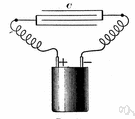# resistivity

(redirected from resistivities)
Also found in: Thesaurus, Medical, Encyclopedia.

## re·sis·tiv·i·ty

(rē′zĭs-tĭv′ĭ-tē)
n. pl. re·sis·tiv·i·ties
1. The capacity for or tendency toward resistance.
2. Electricity An intrinsic property of a material that is measured as its resistance to current per unit length for a uniform cross section.

## resistivity

(ˌriːzɪsˈtɪvɪtɪ)
n
1. (General Physics) the electrical property of a material that determines the resistance of a piece of given dimensions. It is equal to RA/l, where R is the resistance, A the cross-sectional area, and l the length, and is the reciprocal of conductivity. It is measured in ohms. Symbol: ρ Former name: specific resistance
2. the power or capacity to resist; resistance

## re•sis•tiv•i•ty

(ˌri zɪsˈtɪv ɪ ti)

n.
1. the power or property of resistance.
2. electrical resistance measured as a function of a given volume or area.
[1880–85]
ThesaurusAntonymsRelated WordsSynonymsLegend:
 Noun 1resistivity - a material's opposition to the flow of electric current; measured in ohmselectrical phenomenon - a physical phenomenon involving electricityohmage - the ohmic resistance of a conductor
Translations
References in periodicals archive ?
Iso-resistivity/Spatial distribution maps represent the connection between equal electrical apparent resistivities which in turn confirms two-dimensional lateral variations in subsurface lithologies.
The resistivities at an adsorption equilibrium pressure of 0.534 MPa for continuous adsorption and for isovolumetric adsorption are compared in Figure 7, which shows the resistivity changes for the two adsorption methods for the same gas content, for eight isovolumetric gas injection stages.
Some anomalous zones of low electrical resistivity extending below the bottoms of wells CV-4, CV-5, and CV-6 exhibit similar electrical resistivities to those associated with the high quality lithium brine near the bottom of well CV-8.
This method employs a plot of the sum of the apparent resistivities ([SIGMA][[rho].sub.a]) versus depth.
Cucchiaro, "Multilayer ground determination from apparent resistivities and impact on grounding resistances", in Proc.
The interpretation of the VES sounding curve realized close to the borehole indicates that the geoelectric section is composed of six layers: (1) the first three thin near surface layers have high resistivities ranging from 58 to 372 ohm-m and a total thickness of 7.5 m that correspond to top soil and dry alluviums; (2) the 42 m thick fourth layer has an intermediate resistivity of 19 ohm-m and is composed of alluvium; (3) the fifth layer has a relatively high resistivity of 47 ohm-m and also is an aquifer; and (4) the sixth and bottom layer is the conductive geoelectric basement with an average resistivity of 12 ohm-m that is composed mainly of clays.
The three principal resistivities of the half-space are [[rho].sub.x] = [[rho].sup.y ] = 0.5 [OMEGA] x m and [[rho].sub.z]= 2.0 [OMEGA] x m.
The resistivity results show that, for all the soil-cement samples, at freshly prepared state and after one hour of curing time, resistivities are high when compacted on dry side of optimum.
In addition, to evaluate the average effect of fibrous radius R, the static flow resistivities with different fibrous radii are calculated.
Carbon black-filled polymers are used for materials with specific resistivities ranging from 10 to 1018 ohm-cm.
The main purposes of quantitative interpretation of VES are to determine the thickness of the different formations having different resistivities.
However, a distinct difference was observed between their electrical resistivities at room temperature, as shown in Fig.

Site: Follow: Share:
Open / Close